The diagram of a logic circuit is given below. The output F of the circuit is represented by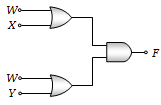(a) W(X+Y)
(b) W.(X.Y)
(c) W+(X.Y)
(d) W+(X+Y)

Concept Questions :-

Logic gates
High Yielding Test Series + Question Bank - NEET 2020

Difficulty Level:

Choose the only false statement from the following-

1.  The resistivity of a semiconductor increases with increase in temperature

2.  Substances with energy gap of the order of 10eV are insulators.

3.  In conductors the valence and conduction bands may over lap

4.  The conductivity of a semiconductor increases with increases in temperature.

Concept Questions :-

Energy band theory

Difficulty Level:

The shows two NAND gates followed by a NOR gate. The system is equivalent to the following logic gate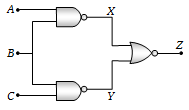(a) OR
(b) AND
(c) NAND
(d) None of these

Concept Questions :-

Logic gates
High Yielding Test Series + Question Bank - NEET 2020

Difficulty Level:

The combination of gates shown below produces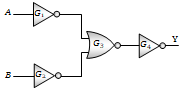(a) AND gate
(b) XOR gate
(c) NOR gate
(d) NAND gate

Concept Questions :-

Logic gates
High Yielding Test Series + Question Bank - NEET 2020

Difficulty Level:

Figure gives a system of logic gates. From the study of truth table it can be found that to produce a high output (1) at R, we must have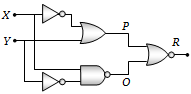(a) X = 0, Y = 1
(b) X = 1, Y = 1
(c) X = 1, Y = 0
(d) X = 0, Y = 0

Concept Questions :-

Logic gates
High Yielding Test Series + Question Bank - NEET 2020

Difficulty Level:

The following configuration of gate is equivalent to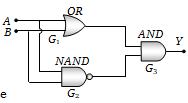(a) NAND
(b) XOR
(c) OR
(d) None of these

Concept Questions :-

Logic gates
High Yielding Test Series + Question Bank - NEET 2020

Difficulty Level:

For the transistor circuit shown below, if $\mathrm{\beta }$ = 100, voltage drop between emitter and base is 0.7 V, then value of ${\mathrm{V}}_{\mathrm{CE}}$ will be :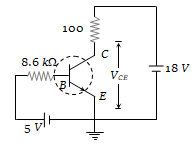(a) 10 V
(b) 5 V
(c) 13 V
(d) 0 V

Concept Questions :-

Transistor
High Yielding Test Series + Question Bank - NEET 2020

Difficulty Level:

In the following circuit, find ${\mathrm{I}}_{1}$ and ${\mathrm{I}}_{2}$.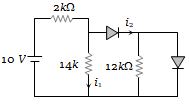(a) 0, 0
(b) 5 mA, 5 mA
(c) 5 mA, 0
(d) 0, 5 mA

Concept Questions :-

Applications of PN junction
High Yielding Test Series + Question Bank - NEET 2020

Difficulty Level:

In the circuit shown in the figure, the maximum output voltage ${\mathrm{V}}_{0}$ is :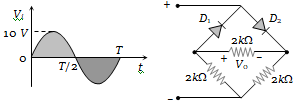1. 0 V                        2. 5 V
3. 10 V                      4. $\frac{5}{\sqrt{2}}$

Concept Questions :-

Rectifier
High Yielding Test Series + Question Bank - NEET 2020

Difficulty Level:

Ge and Si diodes conduct at 0.3 V and 0.7 V respectively. In the following figure if Ge diode connection are reversed, the valve of ${\mathrm{V}}_{0}$ changes by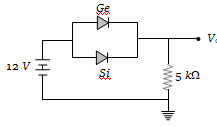(a) 0.2 V                    (b) 0.4 V
(c) 0.6 V                    (d) 0.8 V

Concept Questions :-

PN junction